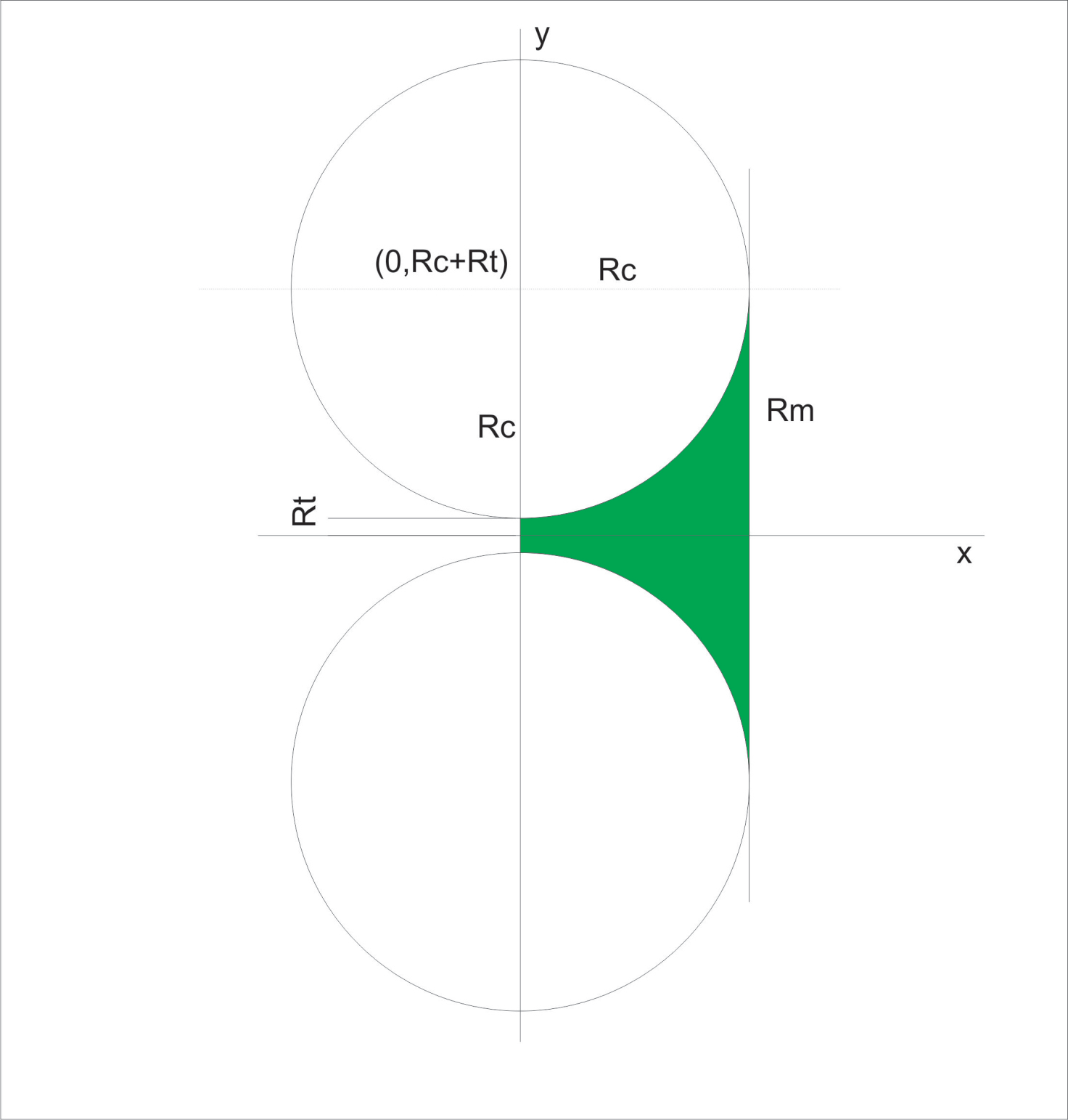## ホーンプロファイル研究 / Horn Profile Study

byPresented here is the way to design and build a quadrant
horn. This horn was born from the need of a simple to build contour. An
exponential or hypex contour can pose problems for an amateur builder
having access just to handheld tools. The quadrant horn can be easily
constructed with the use of a hand operated router.

ここで展示されているのは、Quadrant ホーンの設計と構築の方法です。このホーンは、音調曲線を作るというシンプルな必要性から生まれました。急上昇またはhypexな音調曲線は、小型ツールへのアクセスを持つアマチュア製作者にとっては問題になることがあります。四分円ホーンは、手操作ルーターの使用で簡単に組み立てることができます。

1. The contour calculation:
The horn contour describes an arc of a circle equal in length with
the circumference of a quadrant. Looking at a cross-section along the x
axis we can see that each side defines the outline of a quadrant. The
circles have their centers on the same plane with the horn’s throat so x
coordinate of the center will always be 0.
In practice it can differ as other segments can be added between
the throat and the driver to provide a better loading of the diaphragm.
In order to plot the curve we will look at the fourth quadrant of a
circle. Starting from the circle equation: (x-a)^2+(y-b)^2=R^2. With
(a,b) being the coordinates of the circle’s center and as we stated a=0
then our equation will be x^2+(y-b)^2=R^2.
The reason we chose a=0 is that x will be the axis of expansion for
our curve. This means that for each value of x>=a we will calculate the
value of y and plot the (x,y) point.
Fig. 1. shows a drawing of the horn’s contour. It is important to
notice that the value of b will be calculated knowing the radius of the
throat and either the radius of the circle or the radius of the mouth.
The radius of the mouth can be chosen to meet a desired system’s
dimensions or from the common term in the PA world “mouth frequency” or
the horn’s cutoff frequency.
In fig. 1. we can see that b=Rt+Rc, with Rt= throat radius, Rc=
circle radius. The mouth radius will always equal the value of b,
Rm=Rt+Rc.
Our equation now becomes x^2+(y-Rt-Rc)^2=Rc^2. To determine the
values of y for the fourth quadrant we are interested in the negative
solutions for x=0…Rc. It is important to notice that the value of x
can never exceed the value of Rc.
To plot the curve in positive x,y coordinates the equation is:
y=(Rt+Rc)-sqrt(Rc^2-x^2)
The values of y(x) with x=0…Rc describe just the radius at
distance x of an axisymmetric horn. To determine the diameter or width
we just need to multiply by 2:
D(x)=2*[(Rt+Rc)-sqrt(Rc^2-x^2)]Fig. 1.

2. Modeling
A spreadsheet was developed for calculation of Baffle input
parameters for AxiDriver based on the last equation.
There are some observations that can be easily made looking at
fig.1. As it is, the horn will always have the mouth diameter more than
2 times the length of the horn. This rapid expansion makes it more
suitable for midrange and high frequency usage.
In the next pages are the results of AxiDriver simulations of
different sizes of Rm for the quadrant horn.

As can be seen from BEM simulations, the profile maintains a nice 60 degree directivity all the way to 20kHz.

Attached you can find the spreadsheet to run your own simulations.

quadrant horn spreadsheet

Thank you for visiting / ご覧いただきありがとうございます。